Aidan075121 0 0

↑关注+置顶~ 有趣的不像个技术号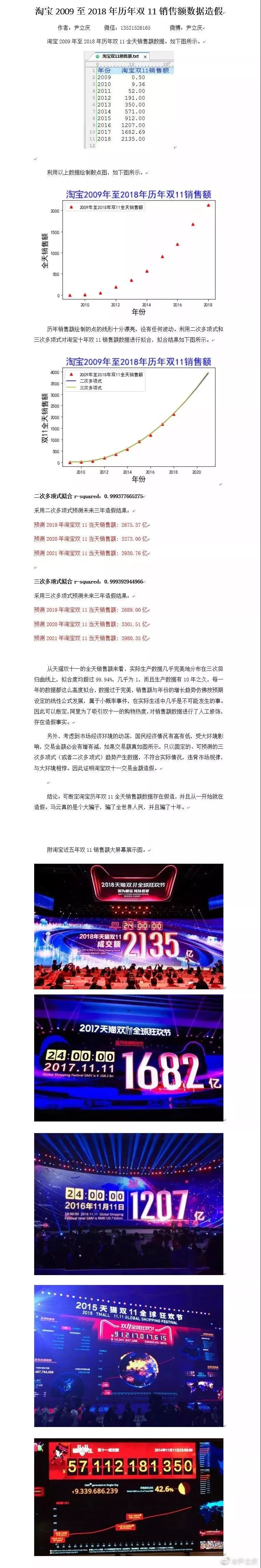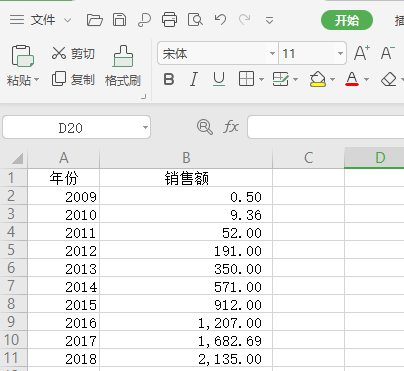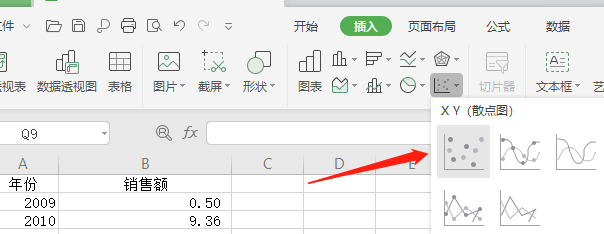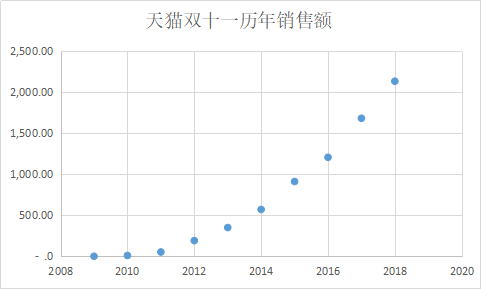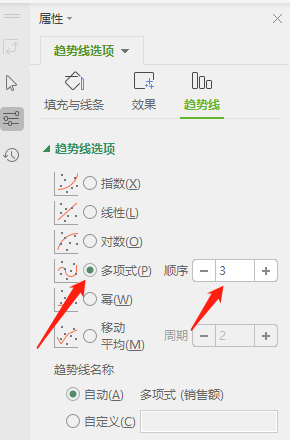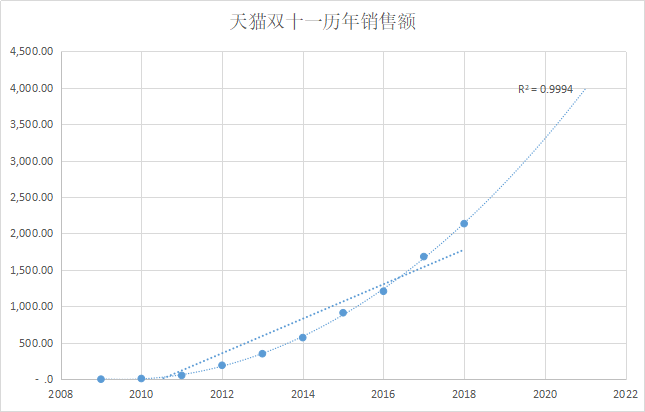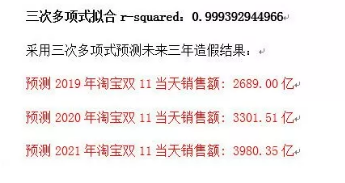over。

import matplotlib.pyplot as plt
import numpy as np

x = np.array([year for year in range(2009,2019)])
y = np.array([0.5,9.36,52,191,352,571,912,1207,1682.69,2135])
z1 = np.polyfit(x, y, 3) # 用3次多项式拟合
p1 = np.poly1d(z1)

yvals=p1(x)
plot1=plt.plot(x, y, '*',label='实际销售额')
plot2=plt.plot(x, yvals, 'r',label='拟合销售额')
plt.xlabel('年份')
plt.ylabel('销售额(亿)')
plt.legend(loc=4) # 指定legend的位置
plt.title('2009-2018淘宝双十一销售额拟合曲线')
plt.figure(figsize=(10, 10))
plt.show()

print('拟合多项式:',p1) #打印拟合多项式
p1 = np.poly1d(z1)
print("-"*40)
print('2019年预测值:',p1(2019))  #打印预测值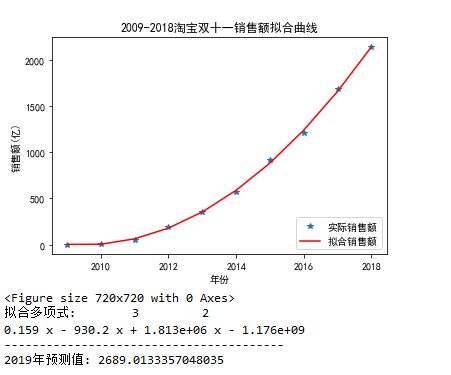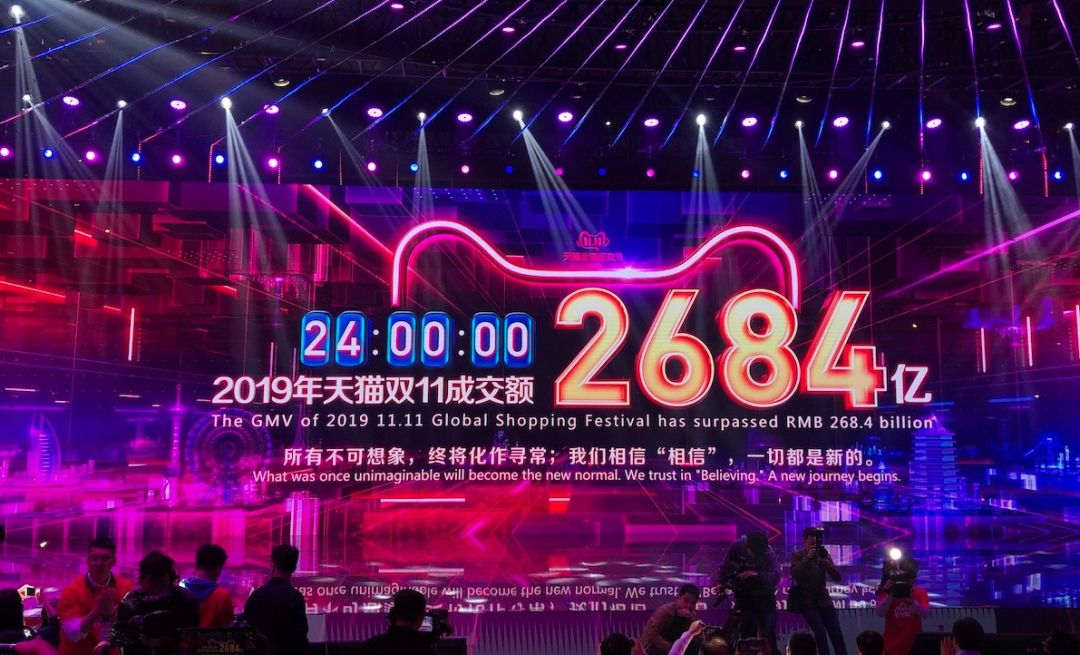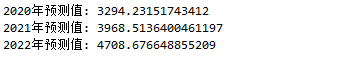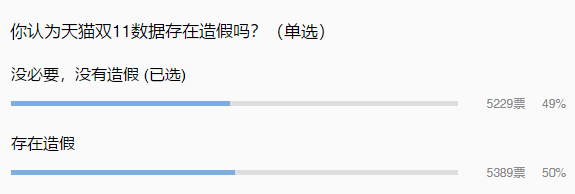End